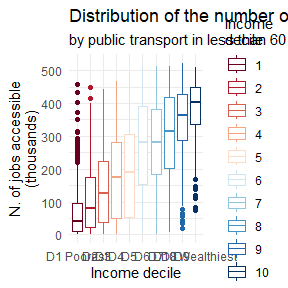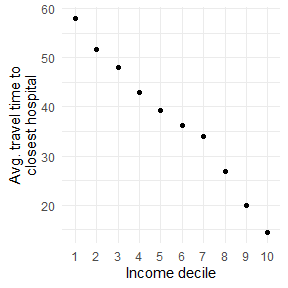#### 2023-05-19

# load libraries
library(aopdata)
library(sf)
#> Warning: package 'sf' was built under R version 4.2.3
library(ggplot2)
#> Warning: package 'ggplot2' was built under R version 4.2.3
library(data.table)
#> Warning: package 'data.table' was built under R version 4.2.3
library(units)
#> Warning: package 'units' was built under R version 4.2.3


mode='public_transport',
peak = TRUE,
year=2019,
showProgress = FALSE)


ggplot() +
geom_boxplot(data=subset(df, !is.na(R003)),
aes(x = factor(R003), y=CMATT60/1000, color=factor(R003))) +
scale_color_brewer(palette = 'RdBu') +
labs(title='Distribution of the number of jobs accessible', color="Income\ndecile",
subtitle='by public transport in less than 60 min. by income decile',
x='Income decile', y="N. of jobs accessible\n(thousands)") +
scale_x_discrete(labels=c("D1 Poorest", paste0('D', 2:9), "D10 Wealthiest")) +
theme_minimal()Palma ratio


# average access of the wealthiest 10%
avg_access_10p_wealthiest <- df[ R003==10, weighted.mean(x=CMATT60, w=P001, na.rm=T)]

# average access of the poorest 40%
avg_access_40p_poorest <- df[ R003<=4, weighted.mean(x=CMATT60, w=P001, na.rm=T)]

# Palma ratio
palma_ratio <- avg_access_10p_wealthiest / avg_access_40p_poorest
palma_ratio
#>  2.609378

This means that:

message( paste0('In less than 60 min. by public transport, the 10% wealthiest population could access on average ', round(palma_ratio,1), ' times more job opportunites than the 40% poorest people') )

Inequality in travel time to closes hospital


# replace Inf travel time with 120
df[, TMISA := fifelse(TMISA==Inf, 120, TMISA)]

# calculate avarage travel time by race
df[, .(average = weighted.mean(x=TMISA, w=P001, na.rm=T),
white   = weighted.mean(x=TMISA, w=P002, na.rm=T),
black   = weighted.mean(x=TMISA, w=P003, na.rm=T))]
#>    average    white    black
#> 1:  37.124 35.75722 43.29197

# calculate avarage travel time by income
temp <- df[, .(average = weighted.mean(x=TMISA, w=P001, na.rm=T)), by=R003]
temp <- na.omit(temp)

ggplot() +
geom_point(data=temp, aes(y=average, x=factor(R003))) +
labs(x='Income decile', y='Avg. travel time to\nclosest hospital') +
theme_minimal()